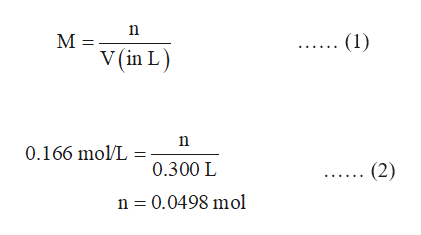# How many grams of ammonium nitrate, NH4NO3, must be dissolved to prepare 300. mL of a 0.166 M aqueous solution of the salt?

Question
94 views

How many grams of ammonium nitrateNH4NO3, must be dissolved to prepare 300. mL of a 0.166 M aqueous solution of the salt?

check_circle

Step 1

The molarity of a solution is given by the equation (1) in which M is the molarity of solution, n is the number of moles of solute and V is the volume of solution in litres.

The volume of solution is 300 mL which is equal to 0.300 L.

The number of moles of ammonium nitrate in the given solution is calculated in the equation (2) by substituting the values of molarity and volume in litres in equation (1).help_outlineImage Transcriptionclose(1) M = v(in L) 0.166 mol/L . 0.300 L (2) n 0.0498 mol fullscreen
Step 2

The number of moles of a substance (n) is given by the equation (3) in which m is the given mass of substance and M is the molar mass.

The mass of ammonium ni...

### Want to see the full answer?

See Solution

#### Want to see this answer and more?

Solutions are written by subject experts who are available 24/7. Questions are typically answered within 1 hour.*

See Solution
*Response times may vary by subject and question.
Tagged in

### Chemistry